Courses

# Test: Arun Sharma Based Level 3: Percentages

## 15 Questions MCQ Test UPSC Prelims Paper 2 CSAT - Quant, Verbal & Decision Making | Test: Arun Sharma Based Level 3: Percentages

Description
This mock test of Test: Arun Sharma Based Level 3: Percentages for UPSC helps you for every UPSC entrance exam. This contains 15 Multiple Choice Questions for UPSC Test: Arun Sharma Based Level 3: Percentages (mcq) to study with solutions a complete question bank. The solved questions answers in this Test: Arun Sharma Based Level 3: Percentages quiz give you a good mix of easy questions and tough questions. UPSC students definitely take this Test: Arun Sharma Based Level 3: Percentages exercise for a better result in the exam. You can find other Test: Arun Sharma Based Level 3: Percentages extra questions, long questions & short questions for UPSC on EduRev as well by searching above.
QUESTION: 1

### X ’s income is 75% of Y ’s income, and X ’s expenditure is 80% of Y ’s expenditure. If X ’s income is 90% o f Y ’s expenditure, find the ratio of X ’s savings to Y ’s savings.

Solution: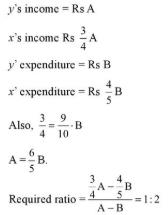QUESTION: 2

### If the length and breadth of a rectangular plot are increased by 20% and 50% respectively, Then the new area is how many times the original area?

Solution:

LetL= 100, B = 100 A= 10000
New length = 120, new breadth =150 New Area = 18000

QUESTION: 3

### There are fifty successive percentage discounts given in a series of 2%, 4%, 6%, 8%... and so on. What is the net discount?

Solution:

Let MP = Rs 100 CP after 1st discount = Rs 98

CP after 2nd discount = Rs 94.08 But discount cannot be more than 100%

QUESTION: 4

Assuming percentage to be true for all the real values, which of the following can be true?

40% of A = 60% of B

i. A > B

ii. B > A

iii. B = A

Solution:

2/5 A = 3/5 B , 2A =3B

QUESTION: 5

Read the passage below and solve the questions based on it.
All students of class X took the pre-board test. One third of the class received B: one fourth received B+: one sixth received B-, and one eighth of the class failed (grade C). Everyone else got A.

Q.

What can be the minimum number of students in class XI

Solution: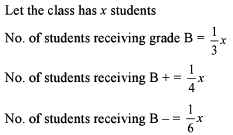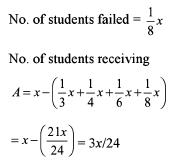For (3x/24) to be an integer, min. value of x = 24.

QUESTION: 6

The cost of an article was Rs.75. The cost was first increased by 20% and later on it was reduced by 20%. The present cost of the article is:

Solution:

Initial Cost = Rs. 75
After 20% increase in the cost, it becomes,
(75 + 20% of 75) = Rs. 90
Now, Cost is decreased by 20%,
So cost will become, (90 - 20% of 90) = Rs. 72
So, present cost is Rs. 72

QUESTION: 7

In the recently concluded referendum in New York, regarding calling back US troops from Iraq, 600 people voted on a referendum, but after some discussion the opponents increased by 150%. The motion was then rejected by a majority two times as great as that which it was formerly passed. How many people voted against the initial referendum?

Solution:

The best way to solve the question is through options.

QUESTION: 8

If 75% of a class of 40 students can sing and only 20% cannot dance, the maximum number of students who can neither sing nor dance is

Solution:

No. of students that can sing = 30

No. of students, that cannot dance = 8

Maximum no. of students that can neither sing nor dance = 8

QUESTION: 9

For admission in a graduation program of Delhi University, 90% of the candidates who appeared for the written test were males and the rest were females, 60% of the males and 80% of the females passed in the written test. What is the total number of students who appeared for the written test, if the total number of passed candidates was 1240?

Solution:

Let total no. of students appeared = x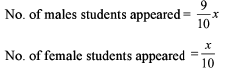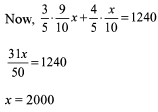QUESTION: 10

60% of the students of a class are boys and in an examination, 80% of the girls scored more than 40 marks (out of a maximum possible 150 marks). If 60% of the total students scored more than 40 marks in the same examination, find the fraction of the boys who scored 40 marks or less.

Solution:

Assume Total no of students = 100
60% of the students are boys, so Boys = 60, Girls = 40
No. of girls who scored more than 40 marks = 80% of girls = 80% of 40 = 32.
No. of students who scored more than 40 marks = 60% of Total Students = 60
Therefore No. of boys who scored more than 40 marks = 60 - 32 = 28
No. of boys who scored less= Total boys – Boys(scored more) = 60 - 28 = 32
Fraction= (scored less)/Total boys = 32/60 =8/15

QUESTION: 11

A community service organisation has raised 80% of the donation that it needed for a new building by receiving an average donation of Rs 400 per person from a group of people. To raise the remaining amount, the organisation now intends approaching another group of people which has two-thirds the number of people as the first group. What should be the average donation per person from the new group?

Solution: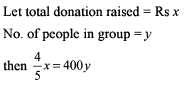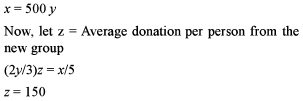QUESTION: 12

In the US opinion polls held during August, 60% of the respondents favoured Walter Mondale while the rest favoured George W. Bush. It was found in September polls that 10% of Mondale supporters switched their preference to Bush, while the same percentage of Bush’s supporters also switched their preference toMondale. What percentage of the electorate should now switch their preference from Mondale to Bush so that they are at par?

Solution:

Let total no. of respondents =100 People favoured Mondale = 60 People favoured blesh = 40 New

No. of people favouring mandale = 58 New No. of people favouring bush = 42

Required percent = 14%

QUESTION: 13

Read the passage below and solve the questions based on it Abhay, Bhaskar. Chitra and Danny work in the same office. Abhay’s Salary is 20% less than Bhaskar's salary, Chitra’s salary is 25% more than Abhay's salary.Danny’s salary is 20% more than Abhay's salary.
Q. If Abhay has a salary of Rs 10,000 per month, who has a salary of Rs 12,500 per month?

Solution:

Salary of Abhay = Rs 10000
Salary of Bhaskar = Rs 12500
Chitra’s salary = Rs 12500
Dany’s Salary = Rs 12000

QUESTION: 14

Read the passage below and solve the questions based on it Abhay, Bhaskar. Chitra and Danny work in the same office. Abhay's salary is 20% less than Bhaskar's salary, Chitra’s salary is 25% more than Abhay's salary. Danny's salary is 20% more than Abhay's salary.
Q. If Chitra received a raise and her new salary is 10% higher than Danny’s, what is her new monthly salary? (use the data from the previous question).

Solution:

Chitra’s New Salary = Rs 13200

QUESTION: 15

The pollution in normal atmosphere is less than 0.01%. Due to leakage of methyl isocyanide (MIC) from a factory the pollution is increased to 20%. If every day 80% of the pollution in the atmosphere is neutralized, in how many days will the atmosphere become normal?

Solution:

The pollution has been increased to 20%.

Every day, 80% pollution is being neutralized, so only 20% of the total pollution remains the next day.
Let x days be needed to bring back the pollution to normal.
Thus, (0.2)x×0.2<0.0001
⇒(0.2)x<0.0005
We have (0.2)4=0.0016 and (0.2)= 0.00032 (can guess the value x on the basis of number of digits after decimal point too)
Hence,minimum of 6 days will be required to bring the pollution back to normal.